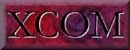### 3. Interpolation and Combination

For the purpose of interpolation with respect to photon energy, the coherent and incoherent scattering cross sections and the total attenuation coefficients are approximated by log-log cubic-spline fits as functions of energy. For the pair-production cross sections, the fitted quantity is the logarithm of the quantity (1-E/E′)3 σPAIR(E), where E is the photon energy, E′ the threshold energy for pair production, and σPAIR(E) is the cross section. The fitting is done separately for pair production in the field of the atomic nucleus (E′ = 1.022 MeV) and in the field of the atomic electrons (E′ = 2.044 MeV).

The combined photoelectric absorption cross section for all shells is similarly interpolated with use of log-log cubic-spline fits, but only at energies above the K-shell absorption edge. Below this energy, interpolation is applied to the logarithm of the photoelectric absorption cross section for each separate shell, fitted as a linear function of the logarithm of the photon energy. The separate fitting for each shell is necessary to avoid the error that would be incurred by interpolating across absorption edges. Linear log-log fitting is equivalent to assuming that the photoelectric cross section is proportional to a power of the photon energy, and was found to provide more satisfactory fits than a log-log cubic-spline fit near the absorption edges.

The interaction coefficients and total attenuation coefficients for compounds are obtained as weighted sums over the corresponding coefficients for elements. XCOM automatically calculates the weight factors, i.e., the fractions by weights of the atomic constituents, from the chemical formula for the compound entered by the user. For mixtures, the user must enter the fractions by weight of the components.

Introduction   |   Database for Elements   |   Interpolation and Combination   |   Using the Program   |   References